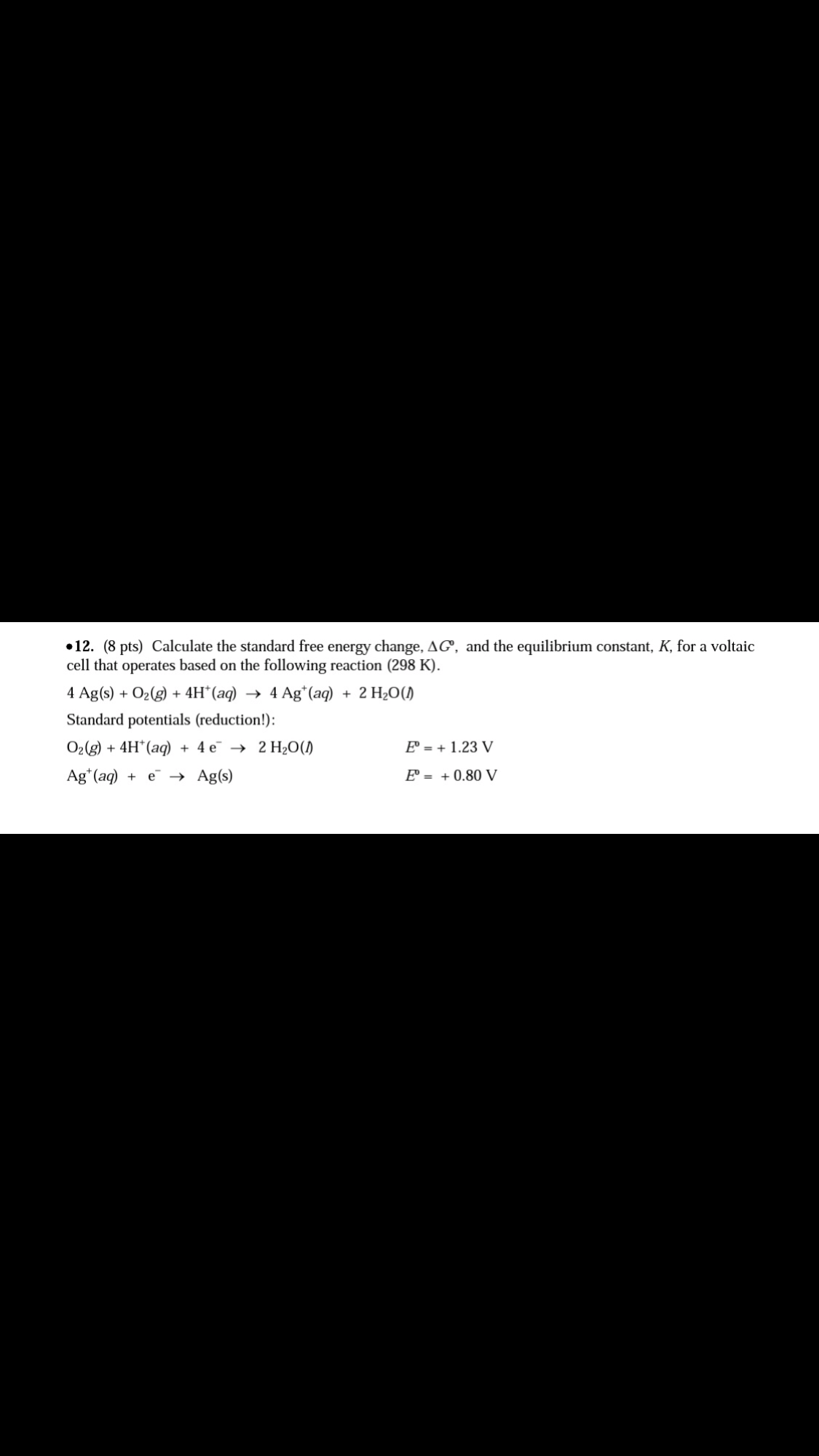# Calculate the standard free energy change, ΔGo, and the equilibrium constant, K, for a voltaic cell that operates based on the following reaction (298 K). 4 Ag(s) + O2(g) + 4H+(aq) → 4Ag+(aq) + 2 H2O(l) Standard potentials (reduction): O2(g) + 4 H+(aq) + 4 e- → 2 H2O (l) Eo = +1.23 V Ag+(aq) + e- → Ag(s) Eo = +0.80 V